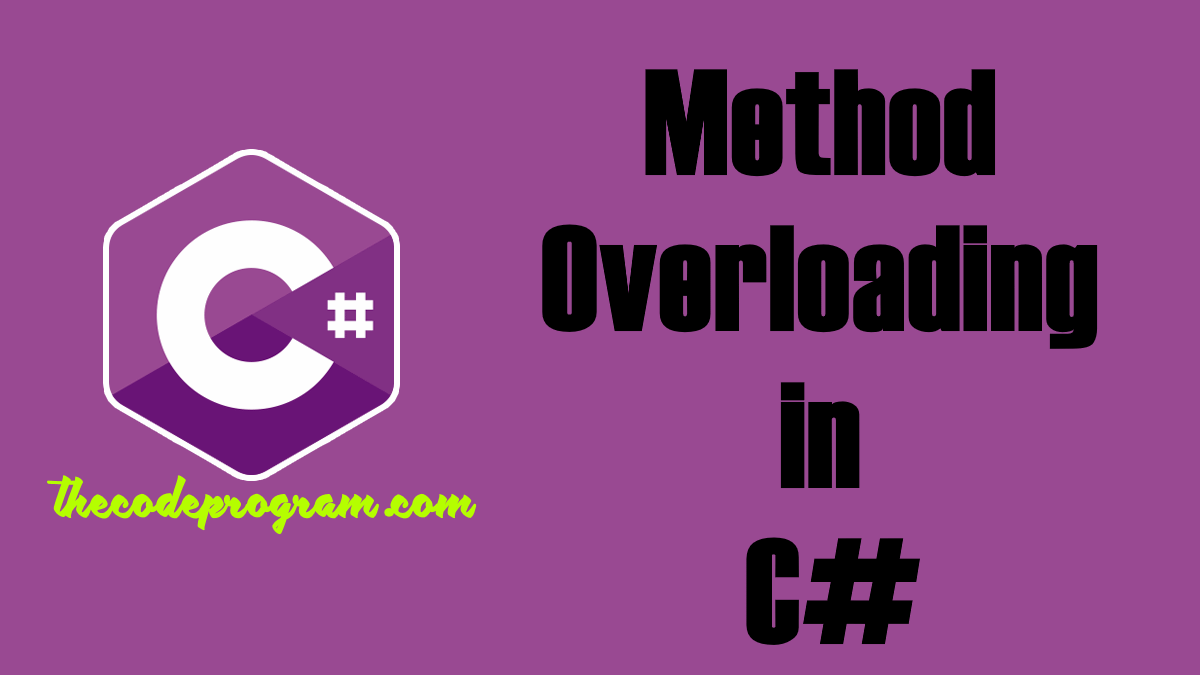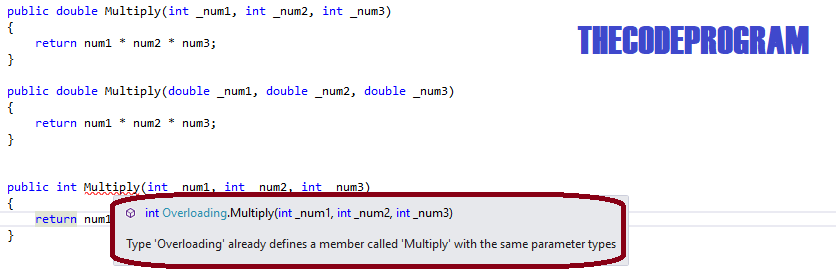Lets begin.

Overloading is a type of polymorphisim. We can define multiple functions with same names and with different parameters and different return types inside the same class. Normally we can not define methods with the same names and same parameters. Purpose of the Method overloading define the same method for the different purposes and with different parameters. You can not define the multiple functions with same parameters and different return types.

We can declare overloaded methods with: Differant types of parameters, Differant types of return type

Now let's write some code:

We can define multiple type constructor methods. We can send the parameters inside the constructor and also we can initiazie the class with null variable values. Here we use the both constructors to initialize the class.

``````
{
public int num1 = 0;
public int num2 = 0;
public int num3 = 0;

//We can define multiple type constructor methods. We can send the parameters inside the constructor
// and also we can initiazie the class with null variable values.
// Here we use the both constructors to initialize the class.

{ }

{
num1 = _num1;
num2 = _num2;
num3 = _num3;
}
}
``````

And we can initiazlie the class as you can seew below.

We can initialize the class like below. We can initialize with parameters and without parameters.

``````
class Program
{
static void Main(string[] args)
{
//We can initialize the class like below.
//We can initialize with parameters and without parameters.
}
}
``````
You can also define methods with multiple types.

Here we declared two methods with same names. One of them has parameters to summarize the other one has ne parameter. Both of them return the same type of data typed value

``````
//Here we declared two methods with same names.
//One of them has parameters to summarize the other one has ne parameter.
//Both of them return the same type of data typed value
public int Sum()
{
return num1 + num2 + num3;
}

public int Sum(int _num1, int _num2, int _num3)
{
return _num1 + _num2 + _num3;
}
``````

We can call overloaded methods like below. If we want to call the method with no parameter it may be good the variables being declared. So I declared the variables and set the values first. or they probably setted before. or we can send the values inside the method as parameters. you see we used the same method names and with parameters and with no parameters.

``````
//We can call overloaded methods like below.
//If we want to call the method with no parameter it may be good the variables being declared.
//So I declared the variables and set the values first. or they probably setted before.
//or we can send the values inside the method as parameters.
//you see we used the same method names and with parameters and with no parameters.
``````

Also we can declare the overloaded methods with different type of return values.

We can also overload the method with different type return value and different type of parameters. One of methods is integer and the other one is double

``````
//We can also overload the method with different type return value
//and different type of parameters. One of methods is integer and the other one is double
public int Multiply()
{
return num1 + num2 + num3;
}

public int Multiply(int _num1, int _num2, int _num3)
{
return num1 + num2 + num3;
}

public double Multiply(double _num1, double _num2, double _num3)
{
return _num1 + _num2 + _num3;
}
``````

We can use the overloaded method. And we used the double parametered function like below. Integer variable type does not take the floated numbers.

``````
//We can use the overloaded method.
//And we used the double parametered function like below.
//Integer variable type does not take the floated numbers.
``````

But if you define the variables with same names and same variable types it will be throw an error like below image. So You can not define methods with same names and differant type return values.I wish you all healthy days.

Burak Hamdi TUFAN.

20/11/2015

23/03/2021

27/04/2023